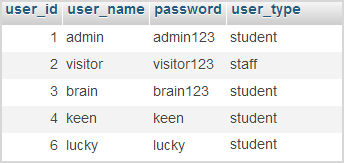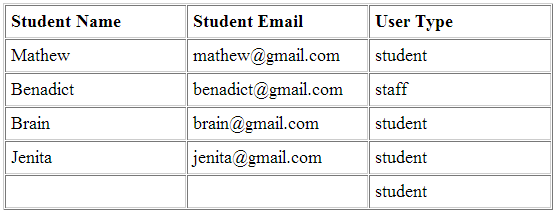# MySQL Right JOIN

Right, JOIN in MySQL is for the same purpose of getting columns from more than one table as like as MySQL Left JOIN we have seen some days before. But, right JOIN differs with the starting point of which table data is preserved to compare another table. It preserves right table data, instead of considering the left table as like as MySQL Left JOIN.Now, we are going to look into how this Right JOIN is performed with the same tables which we took for explaining Left JOIN, to catch the point of difference between these two type of JOIN operations. So, the left JOIN query has been changed by replacing MySQL LEFT keyword as RIGHT as shown below.

```\$query = "SELECT students.student_name, students.student_email, users.user_type FROM students RIGHT JOIN users ON (students.student_id = users.user_id)";
```

By executing this query, then it keeps the right table rows and based on which it searches for the match if any that satisfies the condition specified using ON clause of the above query.

Before starting, let us look into the tables once again for reference as follows.

students Tableusers TableNow, we are going to join these two tables as students RIGHT JOIN users by applying the same condition with ON clause. As we discuss already, RIGHT JOIN considers right table first which is users here in this query. So, it picks user_type for all users based on user_id. And then, it will check for the match if any as specified in ON conditions. That is, if the user_id and student_id of student table are same, then the corresponding value of the columns student_name and student_email will be returned as a query result. Otherwise, it will return a NULL value for left table columns.

On seeing the above tables, all the user_id of users table got matched with the student_id field of another table, except where the user_id is 6 for which the student_name and student_email have no values as shown in the output screenshot below.And, the following PHP program is used to perform right join operations as we discussed above that has slight query keyword modification on the MySQL left JOIN program.

```<?php
\$conn = mysqli_connect("localhost", "root", "test", "blog_samples") or die("Connection Error: " . mysqli_error(\$conn));

\$query = "SELECT students.student_name, students.student_email, users.user_type FROM students RIGHT JOIN users ON (users.user_id = students.student_id)";
\$result = mysqli_query(\$conn,\$query) or die(mysqli_error(\$conn));
?>
<table width="500px" cellpadding="5" cellspacing="1" border="1" style="border-color:#CCCCCC">
<tr>
<td width="33%"><strong>Student Name</strong></td>
<td width="33%"><strong>Student Email</strong></td>
<td width="33%"><strong>User Type</strong></td>
</tr>
<?php
while(\$row = mysqli_fetch_array(\$result)){
?>
<tr>
<td width="33%"><?php echo \$row['student_name']; ?></td>
<td width="33%"><?php echo \$row['student_email']; ?></td>
<td width="33%"><?php echo \$row['user_type']; ?></td>
</tr>
<?php
}
?>
```